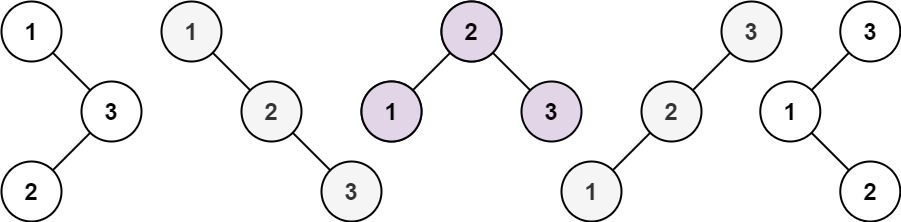# Question

Formatted question description: https://leetcode.ca/all/96.html

Given an integer n, return the number of structurally unique BST's (binary search trees) which has exactly n nodes of unique values from 1 to n.

Example 1:Input: n = 3
Output: 5


Example 2:

Input: n = 1
Output: 1


Constraints:

• 1 <= n <= 19

# Algorithm

Let’s first look at the situation when n = 1, only a single binary search tree can be formed. The situations where n is 1, 2, and 3 are as follows:

			1                        n = 1

2        1                   n = 2
/          \
1            2

1         3     3      2      1           n = 3
\       /     /      / \      \
3     2     1      1   3      2
/     /       \                 \
2     1         2                 3


We assign 1 when n = 0, because the empty tree is also a binary search tree, then the situation when n = 1 can be regarded as the number of left subtrees multiplied by the number of right subtrees, and the left and right subtrees Trees are all empty trees, so 1 by 1 is still 1. Then when n = 2, since both 1 and 2 can be roots, you can calculate them separately and add them together. The case of n = 2 can be calculated by the following formula (where dp[i] represents the number of BST that can be composed of i numbers):

dp =

dp * dp
(If 1 is the root, the left subtree must not exist, and the right subtree can have a number)

+

dp * dp
(If 2 is the root, the left subtree can have a number, and the right subtree must not exist)


In the same way, the calculation method for n = 3 can be written:

dp =
dp * dp
(If 1 is the root, the left subtree must not exist, and the right subtree can have two numbers)

+

dp * dp
(If 2 is the root, the left and right subtrees can each have a number)

+

dp * dp
(If 3 is the root, the left subtree can have two numbers, and the right subtree must not exist)


# Code

• 
public class Unique_Binary_Search_Trees {

public static void main(String[] args) {
Unique_Binary_Search_Trees out = new Unique_Binary_Search_Trees();
Solution s = out.new Solution();

System.out.println(s.numTrees(3));
}

public class Solution {
public int numTrees(int n) {
if (n <= 0) {
return 0;
}

// dp[i] represents the number of BST that can be composed of i numbers
int[] dp = new int[n + 1];
dp = 1; // null is counted as one unique tree

for (int i = 1; i <= n; i++) { // 1...n

for (int j = 1; j <= i; j++) {
// for each fixed n, calculate its sum
dp[i] += dp[j - 1] * dp[i - j];
}
}

return dp[n];
}
}

public class Solution_recursion {
public int numTrees(int n) {
if (n < 0) {
return 0;
}

if (n == 0 || n == 1) {
return 1;
}

int sum = 0;

for (int i = 1; i <= n; i++) {

// 2 conditions: unique && BST. => inorder-visit will generate ordered sequence
// so, if decide root, then left and right can be calculated
// @note: root is "i", left has "i-1" nodes, right has "n - (i - 1) - 1"=="n - i" nodes
sum += numTrees(i - 1) * numTrees(n - i);
}

return sum;
}
}
}

############

class Solution {
public int numTrees(int n) {
int[] dp = new int[n + 1];
dp = 1;
for (int i = 1; i <= n; ++i) {
for (int j = 0; j < i; ++j) {
dp[i] += dp[j] * dp[i - j - 1];
}
}
return dp[n];
}
}

• // OJ: https://leetcode.com/problems/unique-binary-search-trees
// Time: O(N^2)
// Space: O(N)
class Solution {
public:
int numTrees(int n) {
int dp = {[0 ... 1] = 1};
for (int i = 2; i <= n; ++i) {
for (int j = 1; j <= i; ++j) {
dp[i] += dp[j - 1] * dp[i - j];
}
}
return dp[n];
}
};

• class Solution:
def numTrees(self, n: int) -> int:
dp =  * (n + 1)
dp = 1 # e.g, null is counted as one unique tree
for i in range(1, n + 1):
# up to i-1, when j=i-1 meaning left child having i-1 node then i is the root
for j in range(i):
dp[i] += dp[j] * dp[i - j - 1] # -1, not counting root
return dp[-1]

############

class Solution(object):
def _numTrees(self, n):
"""
:type n: int
:rtype: int
"""
dp =  * (n + 1)
dp = dp = 1
for i in range(2, n + 1):
for j in range(1, i + 1):
dp[i] += dp[j - 1] * dp[i - j]
return dp[-1]

def numTrees(self, n):
ans = 1
for i in range(1, n + 1):
ans = ans * (n + i) / i
return ans / (n + 1)


• func numTrees(n int) int {
dp := make([]int, n+1)
dp = 1
for i := 1; i <= n; i++ {
for j := 0; j < i; j++ {
dp[i] += dp[j] * dp[i-j-1]
}
}
return dp[n]
}